# h-tree-cli

1.2.0 • Public • Published

# h-tree-cli

Print the H-Tree Fractal to the console!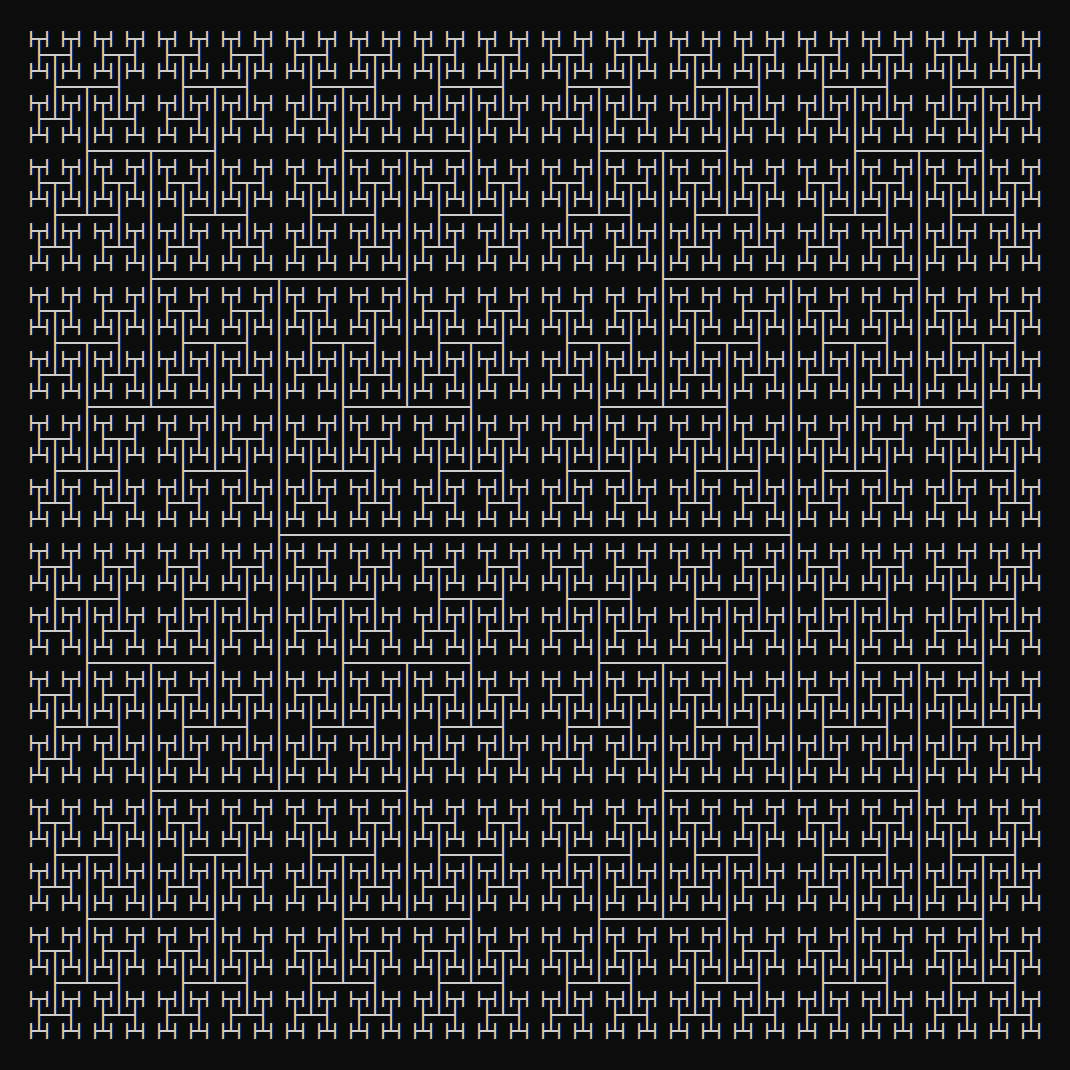Why the console? Because it's the cool way.

See All Fractals in the fractals-cli project.

## Usage

### Via `npx`:

``````\$ npx h-tree-cli <n>
\$ npx h-tree-cli <n> [size] [options]
``````

where `n >= 0` and `size >= n` (if provided).

### Via Global Install

``````\$ npm install --global h-tree-cli
\$ h-tree-cli <n>
\$ h-tree-cli <n> [size] [options]
``````

where `n >= 0` and `size >= n` (if provided).

### Via Import

``````\$ npm install h-tree-cli
``````

then:

``````const h_tree = require('h-tree-cli');
console.log(h_tree.create(<n>);
console.log(h_tree.create(<n>, {
size: <number>,
line: <bold|double|standard>
});
``````

The config params are optional.

## Options

### Recursive Step

``````\$ h-tree-cli <n>
``````

The first param `<n>` is the recursive step. `<n>` should be an integer greater than or equal to 0.

#### Examples:

``````\$ h-tree-cli 7
``````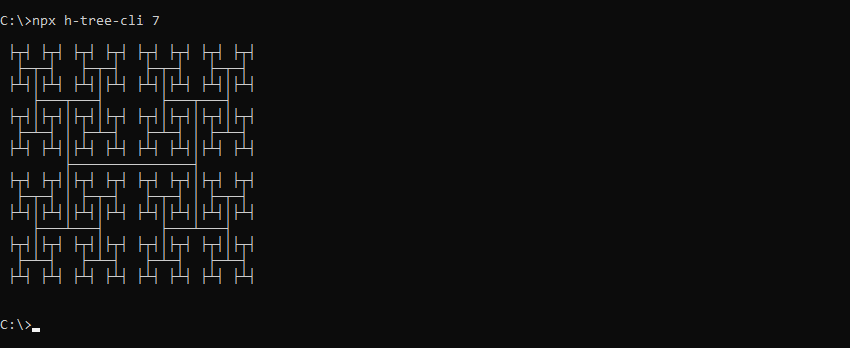``````\$ h-tree-cli 9
``````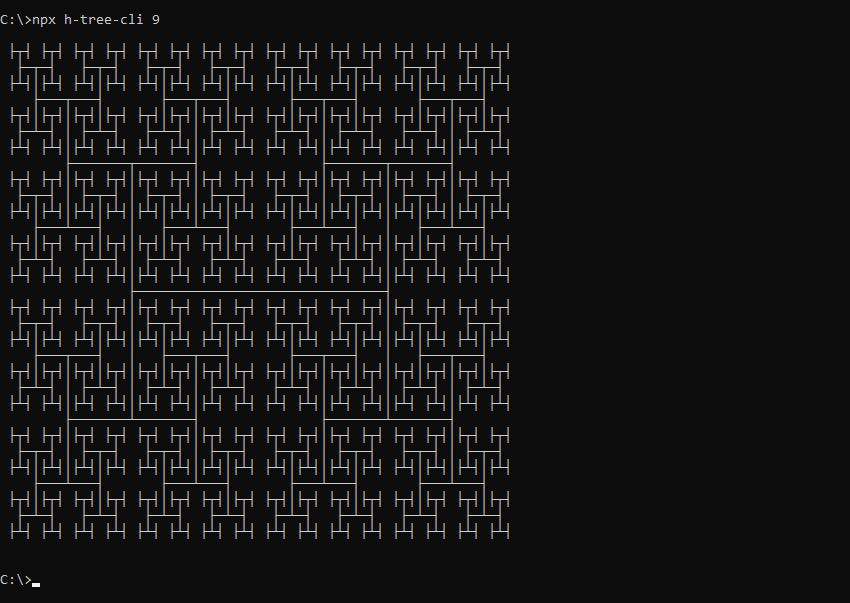### Size

``````\$ h-tree-cli <n> [size]
``````

The optional `[size]` param allows the H-Tree fractal to be drawn at larger sizes. `[size]` should be an integer greater than or equal to `<n>`. Including size will draw a H-Tree fractal of `<n>` recursive steps the size of a H-Tree fractal with `[size]` recursive steps.

#### Example:

``````\$ h-tree-cli 6 9
``````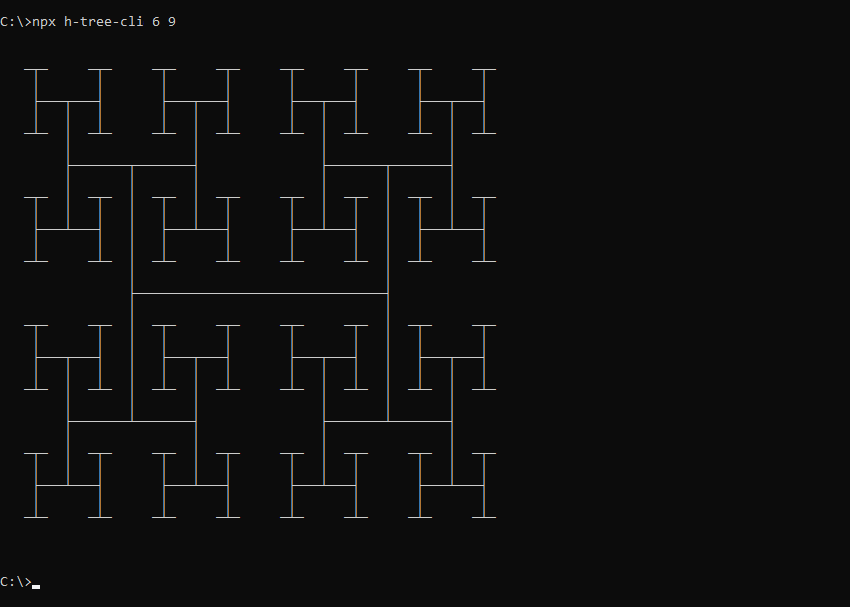### Line Type

``````\$ h-tree-cli <n> --line=<bold|double|standard>
``````

The optional `--line` param draws the H-Tree fractal using different line types. Supported values:

• `bold`: Draw using bold lines
• `double`: Draw using double lines
• `standard`: Draw using standard lines (default)

#### Examples:

``````\$ h-tree-cli 9 --line=bold
``````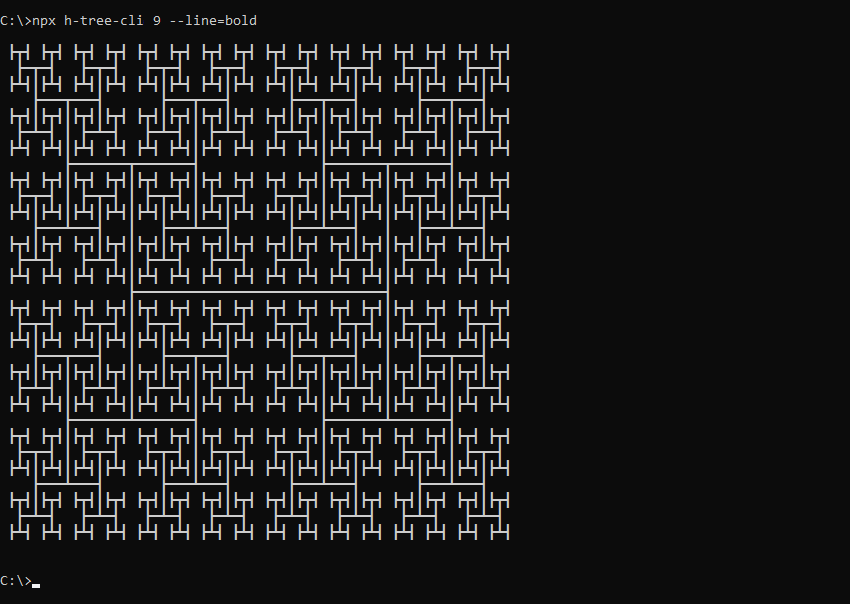``````\$ h-tree-cli 9 --line=double
``````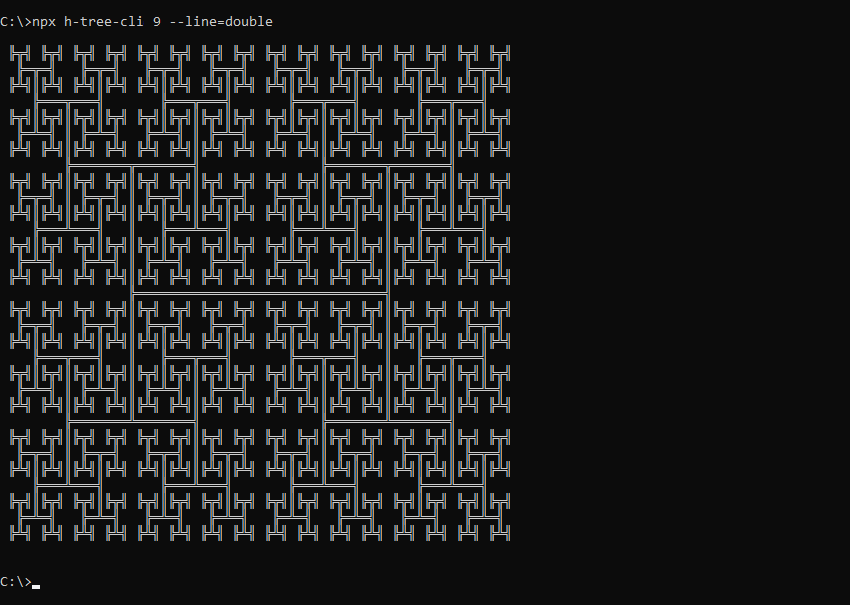## Package Sidebar

### Install

`npm i h-tree-cli`

### Repository

github.com/spirometaxas/h-tree-cli

### Homepage

spirometaxas.com/projects/fractals-cli

0

1.2.0

MIT

15.3 kB

5